Courses

# Test: Power Electronics - 4

## 10 Questions MCQ Test GATE Electrical Engineering (EE) 2022 Mock Test Series | Test: Power Electronics - 4

Description
This mock test of Test: Power Electronics - 4 for Electrical Engineering (EE) helps you for every Electrical Engineering (EE) entrance exam. This contains 10 Multiple Choice Questions for Electrical Engineering (EE) Test: Power Electronics - 4 (mcq) to study with solutions a complete question bank. The solved questions answers in this Test: Power Electronics - 4 quiz give you a good mix of easy questions and tough questions. Electrical Engineering (EE) students definitely take this Test: Power Electronics - 4 exercise for a better result in the exam. You can find other Test: Power Electronics - 4 extra questions, long questions & short questions for Electrical Engineering (EE) on EduRev as well by searching above.
QUESTION: 1

### Which of the following are the advantages of freewheeling of load current in phase – controlled converters? 1. It reduces the reactive power demand of the converter 2. It improves the displacement factor 3. It reduces the generated ripple voltage on the DC side of an SCR phase controlled converter 4. It improves the input PF. Select the correct answer using the codes given below

Solution: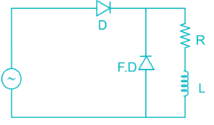Advantage of freewheeling diode

1. It prevents the output voltage from becoming negative

2. System efficiency is improved because energy stored in L is transferred to load R through FD.

3. Load current waveform is more smooth.

4. It reduces the reactive power demand of the converter.

5. It improves the displacement factor.

6. It improves distortion factor.

7. It improves the input PF.

*Answer can only contain numeric values
QUESTION: 2

### A battery is charged by a single phase half wave diode rectifier. The supply voltage is 220 V (rms), 50 Hz and the battery emf is constant at 80 V. then PIV rating of the diode should be___ (in V)

Solution:

PIV = Vm + E = 220√2 + 80 = 3 91.13 V

QUESTION: 3

### A single phase full – wave half controlled bridge converter feeds an inductive load. The two SCRs in the converter are connected to a common DC bus. The converter has to have a freewheeling diode.

Solution:

Single phase full wave half controlled bridge converter feeds an Inductive load. The two SCRs in the converter are connected to a common dc bus. The converter has to have freewheeling diode because the converter does not provide for freewheeling for high value of triggering angles

QUESTION: 4

If the commutation angle of a diode rectifier (due to source inductive effect) is μ, then the inductive voltage regulation will be

Solution:

Voltage regulation =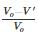for single phase full converter bridge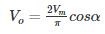due to source inductance effect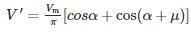Where μ is overlapping angle and α is firing angle
for α = 0, V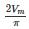and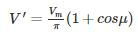Voltage regulation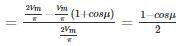QUESTION: 5

A single phase controlled rectifier bridge consists of one SCR and three diode as shown in figure find the waveform of current through T1.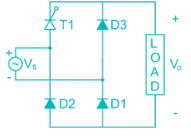Solution: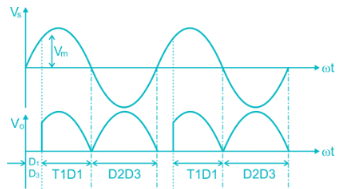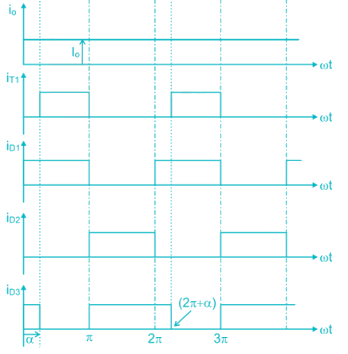*Answer can only contain numeric values
QUESTION: 6

1 ϕ full bridge converter with freewheeling diode feeds an inductive load. Load resistance is 15 Ω and it has a large inductance providing constant and ripple free dc current input to converter from ideal 220 V, 50 Hz single phase source. For a firing angle of 30°, the value of output current is _________A.

Solution:

the average output voltage for single phase with a freewheeling diode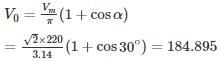The average output current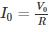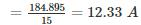*Answer can only contain numeric values
QUESTION: 7

A single phase full converter is connected to an AC supply of 300 sin 314 t V. It operates at firing angle α = 30° the load current maintained constant at 5A and the load voltage 120 V. Calculate source inductance (in mH).

Solution: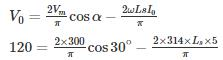120 × 3.14 = 600 cos 30° - 3140 Ls

376.8 = 519.61 – 3140 Ls

-142.82 = -3140 Ls

Ls = 45.48 mH

QUESTION: 8

A single-phase full bridge diode rectifier is supplied from 220 V, 50 Hz source the load consist of R = 15 Ω and a large Inductance so as to render the load current constant determine RMS value of diode current and supply P.F.

Solution:

Average value of output voltage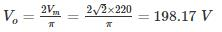Average value of output current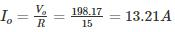R.M.S value of diode current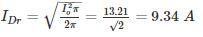Load power = VoIo = 198.17 × 13.21 = 2617.83 W

Input power = VsIs cos ϕ

220 × 13.21 cos ϕ = 2617.83

cos ϕ = 0.9

*Answer can only contain numeric values
QUESTION: 9

A single phase semiconductor, connected to 220 V 50 Hz Is feeding load R = 15 Ω in series with a large inductance that makes the load current ripple free for firing angle of 60°, calculate the Rectification efficiency ______ in %.

Solution:

Concept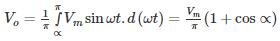R.M.S value of output voltage is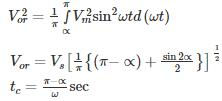Calculation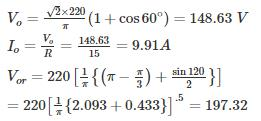The load current is Ripple free

∴ Ior = 197.32/15 = 13.15 A

Pdc = VoIo = 148.63 × 9.91 = 1472.93 W

Pac = VorIor = 197.32 × 13.15 = 2595.46 W

Rectification efficiency =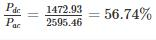*Answer can only contain numeric values
QUESTION: 10

Find out the ratio of the thyristor to diode RMS current rating in the signal phase half controlled converter for firing angle α = 30°. Assume ripple free contains conduction.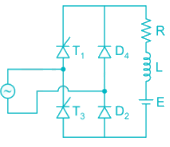Solution:

Conduction diagram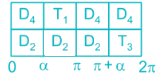From the conduction diagram the thyrsistor conduct for (π – α) radian while the diodes conducts for (π + α) radians. Since the load current is constant.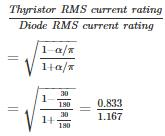= 0.845